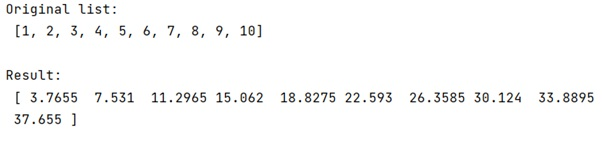# How to multiply each element in a list by a number?

Learn, how to multiply each element in a list by a number in Python?
Submitted by Pranit Sharma, on February 14, 2023

NumPy is an abbreviated form of Numerical Python. It is used for different types of scientific operations in python. Numpy is a vast library in python which is used for almost every kind of scientific or mathematical operation. It is itself an array which is a collection of various methods and functions for processing the arrays.

## Multiplying each element in a list by a number

Suppose that we are given a list and we need to multiply each element of this list with a specific number.

For this purpose, we will simply convert the list into a numpy array using the array object and then we will simply product the array and the number with each other.

Let us understand with the help of an example,

## Python code to multiply each element in a list by a number

```# Import numpy
import numpy as np

# Creating a list
l = [1,2,3,4,5,6,7,8,9,10]

# Display original list
print("Original list:\n",l,"\n")

# Defining a number
n = 3.7655

# Converting list into numpy array
arr = np.array(l)

# Multiplying list elements with n
res = arr*n

# Display the result
print("Result:\n",res,"\n")
```

Output: